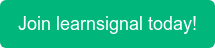##### What are Forward Rates?

The future spot rates implied by today's spot rates are forward rates. Consider the following scenario: the offered one-year rate is 3%, and the offered two-year rate is 4%. (both with annual compounding). We can estimate that the rate provided for the second year is 5%. This is because averaging 3 per cent for the first year with 5 per cent for the second year results in a total of 4 per cent for the two years.

###### Example of Forward Rates:

Suppose F is the forward rate for the second year. The forward rate is such that USD

100, if invested at 3% for the first year and at a rate of F for the

second year, gives the same outcome as 4% for two years. This

means that:

100 x 1.03 x (1 + F) = 100 x 1.042^2

so that:

F=1.042^2/1.03= 1.03 - 1 = 0.0501

or 5.01%.

This is close to the 5% given by our approximate argument. However, it is not the same because there are non-linearities when rates are expressed with annual compounding.

When rates are expressed with semi-annual compounding (as is frequently the case in fixed-income markets), an extension of this analysis shows that the forward rate per six months for six months starting at the time T is

(1 + R2/ 2)t+0-5

(1 + R,/2)T (10.5)

where and R2 are the spot rates for maturities T and T + 0.5

(respectively) with semi-annual compounding. Thus, the annualised forward rate expressed with semi-annual compounding is twice this.

###### Why are forward rates important?

Knowing the future rate is valuable regardless of whatever version is used. It allows the investor to choose the investment option (purchasing one T-bill or two) that offers the best chance of profit.

Topics: ACCA, CIMA, CPD, AAT, FRM•### Central Counterparties

•### Country Risk

•### Convexity Formula

•### Clean and Dirty Price

•### Basic Indicator Approach

•### ACCA Exam Fees 2022: Everything You Need to Know

•### ACCA Exam Dates for 2022

•### How Difficult is Passing ACCA? An Honest Review

•### 7 of the Best YouTube Channels for Accounting Students

•